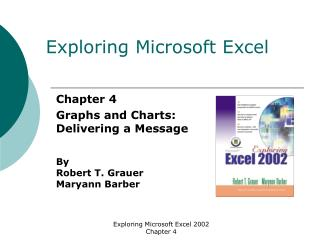Download PresentationExploring Microsoft Excel

# Exploring Microsoft Excel - PowerPoint PPT PresentationDownload Presentation## Exploring Microsoft Excel

- - - - - - - - - - - - - - - - - - - - - - - - - - - E N D - - - - - - - - - - - - - - - - - - - - - - - - - - -
##### Presentation Transcript

1. Exploring Microsoft Excel Chapter 4 Graphs and Charts: Delivering a Message By Robert T. Grauer Maryann Barber Exploring Microsoft Excel 2002 Chapter 4

2. Objectives (1 of 2) • Know advantages and disadvantages of different chart types • Distinguish between an embedded chart and one in a separate chart sheet • Use the ChartWizard • Use Drawing toolbar to enhance chart with lines, 3D shapes and objects Exploring Microsoft Excel 2002 Chapter 4

3. Objectives (2 of 2) • Differentiate between data series specified in rows versus ones in columns • Create a compound document consisting of word processing, worksheet and a chart Exploring Microsoft Excel 2002 Chapter 4

4. Overview • Business graphics one of most exciting Windows applications • Determine message of chart • Create charts using Chart Wizard • Plot multiple data sets on a single chart • Dynamically link a chart to a memo Exploring Microsoft Excel 2002 Chapter 4

5. What is a Chart? • A graphic representation of data in a worksheet • Based on descriptive (text) entries called category labels and numeric values called data points • Data series: a grouping of data points Exploring Microsoft Excel 2002 Chapter 4

6. Chart Types • Always remember to keep it simple and clear • Pie and Exploded pie charts are effective for displaying proportional relationships • Column charts are used for displaying numbers • Bar charts show numbers horizontally Exploring Microsoft Excel 2002 Chapter 4

7. Pie Charts Exploring Microsoft Excel 2002 Chapter 4

8. Column Charts Exploring Microsoft Excel 2002 Chapter 4

9. Creating A Chart • Select the cells containing your labels and data points • Use the Chart Wizard a four step process • Step one choose a chart type • Step two review data series • Step three final touches • Step four where to store Exploring Microsoft Excel 2002 Chapter 4

10. Chart Wizard Exploring Microsoft Excel 2002 Chapter 4

11. Finishing the Chart • Embedding in a worksheet or separate chart sheet • Enhance with Drawing toolbar Exploring Microsoft Excel 2002 Chapter 4

12. Hands-On Exercise 1 • Objective: To create and modify a chart; to embed a chart within a worksheet; to enhance a chart • The AutoSum command • Start the Chart Wizard • Move and Size the chart • Change the worksheet • Change the chart type • Create the second chart • Add a text box • Create a 3-D Shape Exploring Microsoft Excel 2002 Chapter 4

13. Using the Drawing toolbar Exploring Microsoft Excel 2002 Chapter 4

14. Multiple Data Series • Decide what message to convey with the chart • What do you want to emphasize? • Rows versus columns • If data series are in rows the Chart Wizard will use first row for X axis and use first column for legend text • If data series are in columns the Chart Wizard will use first column for X axis and use first row for legend text Exploring Microsoft Excel 2002 Chapter 4

15. Stacked Column Charts • A column chart that depicts totals by category instead of each individual data point • Useful when you want to compare totals by category Exploring Microsoft Excel 2002 Chapter 4

16. Hands-On Exercise 2 • Objective: To plot multiple data series in the same chart; to differentiate between data series in rows and columns; to create multiple charts associated with the same worksheet • Rename the worksheets • The Office Assistant • View the data series • Copy the chart sheet • Change the source data • Change the chart type Exploring Microsoft Excel 2002 Chapter 4

17. Using Multiple Data Series Exploring Microsoft Excel 2002 Chapter 4

18. Object Linking and Embedding • Create a document in one application that contains objects from another application • For example, contain a Word document that contains objects from an Excel worksheet • Embedded object is stored in the document • an Excel chart becomes part of the Word document • Linked object is stored in its own file • any change in this file is automatically reflected in the main document • Multitasking – the ability to have more than one application open at the same time Exploring Microsoft Excel 2002 Chapter 4

19. Hands-On Exercise 3 • Objective: To create a compound document consisting of a memo, worksheet, and chart • Open the software sales document • Copy the worksheet • Create the link • Copy the chart • Add the chart • Modify the worksheet • Update the links • The finishing touches Exploring Microsoft Excel 2002 Chapter 4

20. Summary (1 of 2) • Charts are an easy to understand way to show data • Easiest way to create charts is with the Chart Wizard • Charts can be embedded or stored in a separate file • Multiple data series may be stored in rows & columns Exploring Microsoft Excel 2002 Chapter 4

21. Summary (2 of 2) • OLE enables creation of compound documents • Embedding means storing an object within the compound document • Linking means storing the object in its own file, and the compound document uses this file Exploring Microsoft Excel 2002 Chapter 4